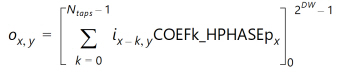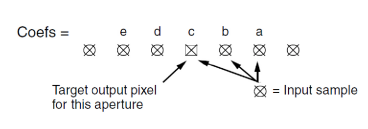# Convert 4:2:2 to 4:4:4 - 2.4 English

## Video Processing Subsystem Product Guide (PG231)

Document ID
PG231
Release Date
2022-10-19
Version
2.4 English

This conversion is a 1:2 horizontal interpolation operation, implemented using a two-phase polyphase FIR filter. One of the two output pixels is co-sited with one of the input sample. The ideal output is achieved simply by replicating this input sample.

To evaluate output pixel o x,y, the FIR filter convolves COEFk_HPHASEp x , where k is the coefficient index, i x,y are pixels from the input image, p x is the interpolation phase (0 or 1, depending on x) and [ ] M m represents rounding with clipping at M, and clamping at m. DW is the Data Width or number of bits per video component. N taps is the number of filter taps.

Equation 3-10In phase 1, COEF00_HPHASE1 is the coefficient applied to the most recent input sample in the filter aperture. This Figure illustrates coefficient use for a four tap filter example, with simplified nomenclature a= COEF00_HPHASE1, b= COEF01_HPHASE1, c=COEF02_HPHASE1, and d= COEF03_HPHASE1.

 X-Ref Target - Figure 3-13The predefined filters replicate the input sample for Phase 0. The Phase 1 filter is [0.5 0.5].